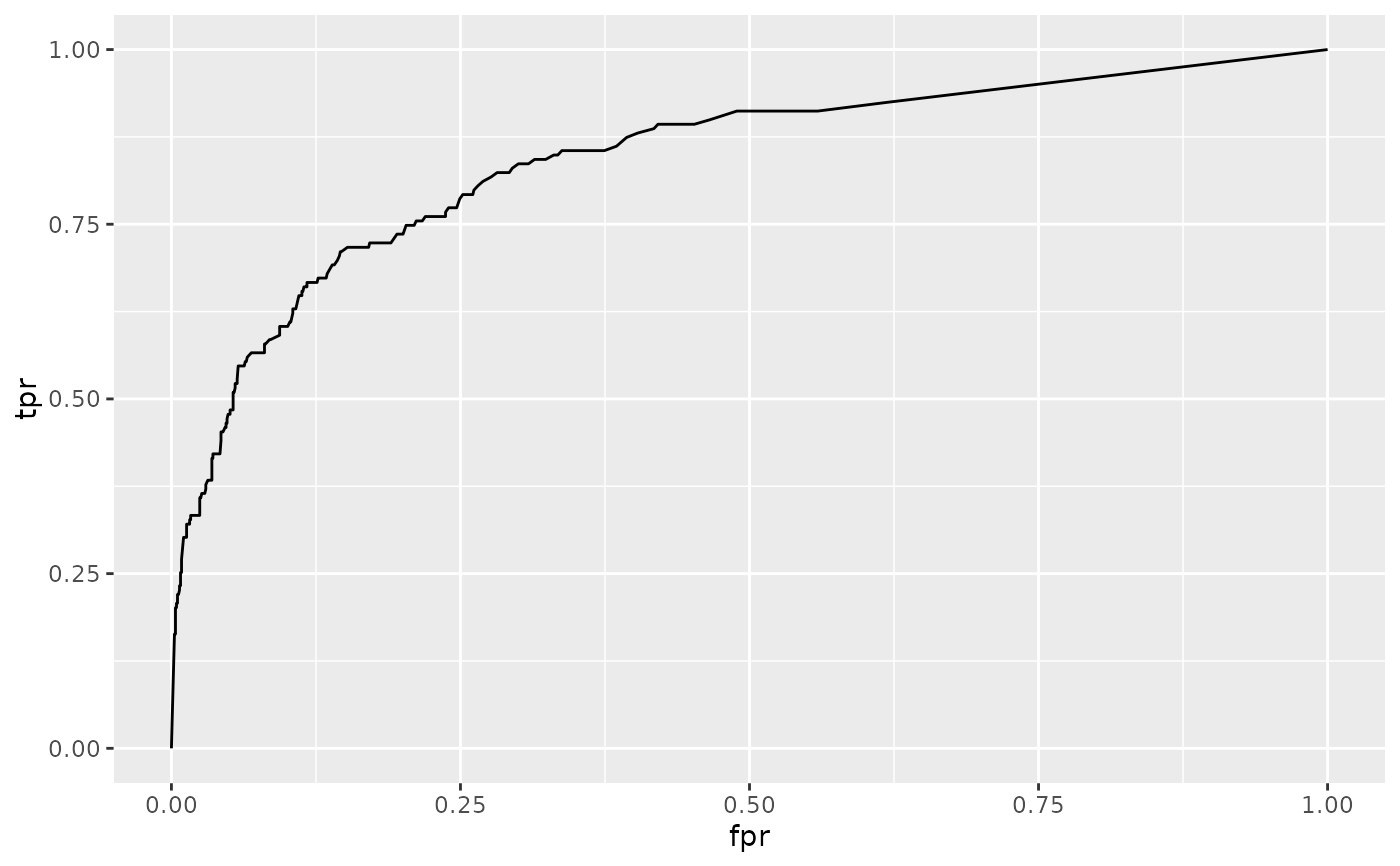Tidy summarizes information about the components of a model. A model component might be a single term in a regression, a single hypothesis, a cluster, or a class. Exactly what tidy considers to be a model component varies across models but is usually self-evident. If a model has several distinct types of components, you will need to specify which components to return.

## Usage

# S3 method for roc
tidy(x, ...)

## Arguments

x

An roc object returned from a call to AUC::roc().

...

Additional arguments. Not used. Needed to match generic signature only. Cautionary note: Misspelled arguments will be absorbed in ..., where they will be ignored. If the misspelled argument has a default value, the default value will be used. For example, if you pass conf.lvel = 0.9, all computation will proceed using conf.level = 0.95. Two exceptions here are:

• tidy() methods will warn when supplied an exponentiate argument if it will be ignored.

• augment() methods will warn when supplied a newdata argument if it will be ignored.

tidy(), AUC::roc()

## Value

A tibble::tibble() with columns:

cutoff

The cutoff used for classification. Observations with predicted probabilities above this value were assigned class 1, and observations with predicted probabilities below this value were assigned class 0.

fpr

False positive rate.

tpr

The true positive rate at the given cutoff.

## Examples


# load libraries for models and data
library(AUC)
#> AUC 0.3.2
#> Type AUCNews() to see the change log and ?AUC to get an overview.
#>
#> Attaching package: ‘AUC’
#> The following objects are masked from ‘package:caret’:
#>
#>     sensitivity, specificity

data(churn)

# fit model
r <- roc(churn$predictions, churn$labels)

# summarize with tidiers + visualization
td <- tidy(r)
td
#> # A tibble: 220 × 3
#>    cutoff     fpr   tpr
#>     <dbl>   <dbl> <dbl>
#>  1  1     0       0
#>  2  1     0.00262 0.164
#>  3  0.972 0.00350 0.164
#>  4  0.968 0.00350 0.182
#>  5  0.964 0.00350 0.189
#>  6  0.96  0.00350 0.201
#>  7  0.932 0.00437 0.201
#>  8  0.91  0.00437 0.208
#>  9  0.908 0.00525 0.208
#> 10  0.902 0.00525 0.214
#> # ℹ 210 more rows

library(ggplot2)

ggplot(td, aes(fpr, tpr)) +
geom_line()# compare the ROC curves for two prediction algorithms
library(dplyr)
library(tidyr)

rocs <- churn %>%
pivot_longer(contains("predictions"),
names_to = "algorithm",
values_to = "value"
) %>%
nest(data = -algorithm) %>%
mutate(tidy_roc = purrr::map(data, ~ tidy(roc(.x$value, .x$labels)))) %>%
unnest(tidy_roc)

ggplot(rocs, aes(fpr, tpr, color = algorithm)) +
geom_line()